# Python全栈工程师（递归函数、闭包）

2018/07/17 00:41

ParisGabriel

每天坚持手写  一天一篇  决定坚持几年 全栈工程师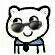Python人工智能从入门到精通

是指用一系列函数解决问题
每一个函数完成细小的功能，一系列函数的任意组合可以完成
大问题
函数仅接受输入并产生输入，不包含任何影响输出的内部 状态

如果一个函数的输入参数一定，则返回结果必须一定
的函数称为可重用函数

可重入和不可重入就是访问除局部变量以外的变量

def 创建函数最好不要访问局部作用域以外的变量，这样可以保证
结果的唯一性（可重入性）

满足下列条件之一的就是高阶函数
1.函数接受一个或多个函数作用参数传入
1.函数返回一个函数

map，filter，sorted

map(func, *iterables)：
用函数和对可迭代对象中的每一个元素作为参数计算出新的可迭代对象,
当最短的一个可迭代对象不再提供数据时此可迭代对象生成结束

filter(function, iterable)：
筛选可迭代对象iterable中的数据,返回一个可迭代器对象，此可迭代对象
将对iterable进行筛选.函数function 将对iterable中的每个元素进行求值，
返回False则将此数据丢弃，返回True，则保留此数据
sorted(iterable, key=None, reverse=False)：
将原可迭代对象的数据进行排序，生成排序后的列表iterable 可迭代对象
key 函数是用来提供一个值,这个值将作为排序的依据reverse 标志用来设
置是否降序排序

range（）
map（）
filter（）
reverse（）

函数直接调用或间接的调用自身
直接调用自己：
def f（）：
f（）
间接调用自己：
def fa（）：
fb（）
def fb（）：
fa（）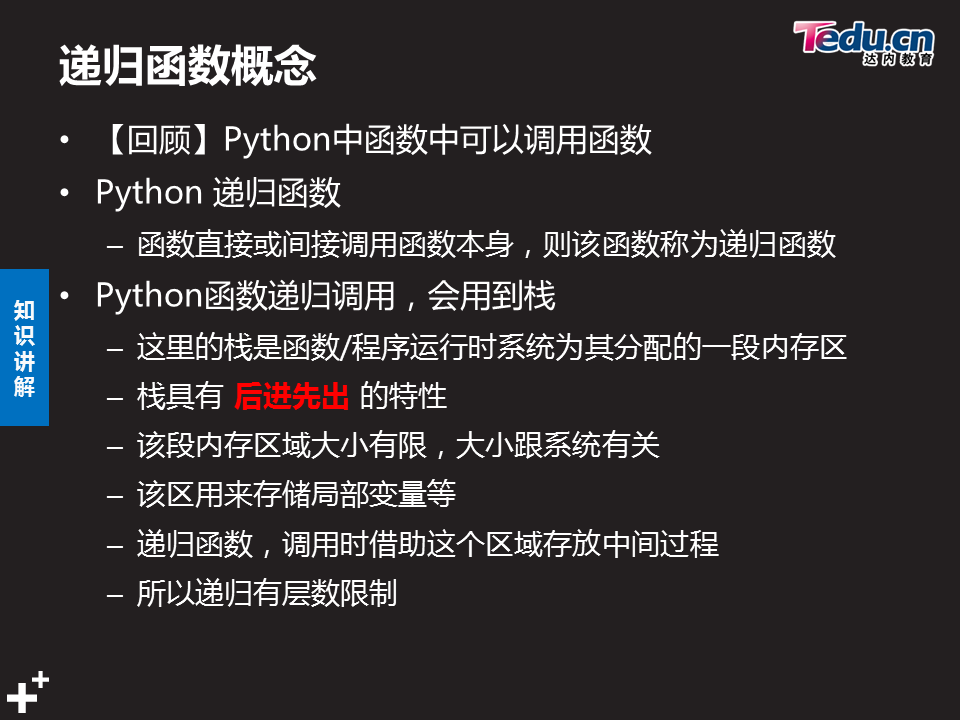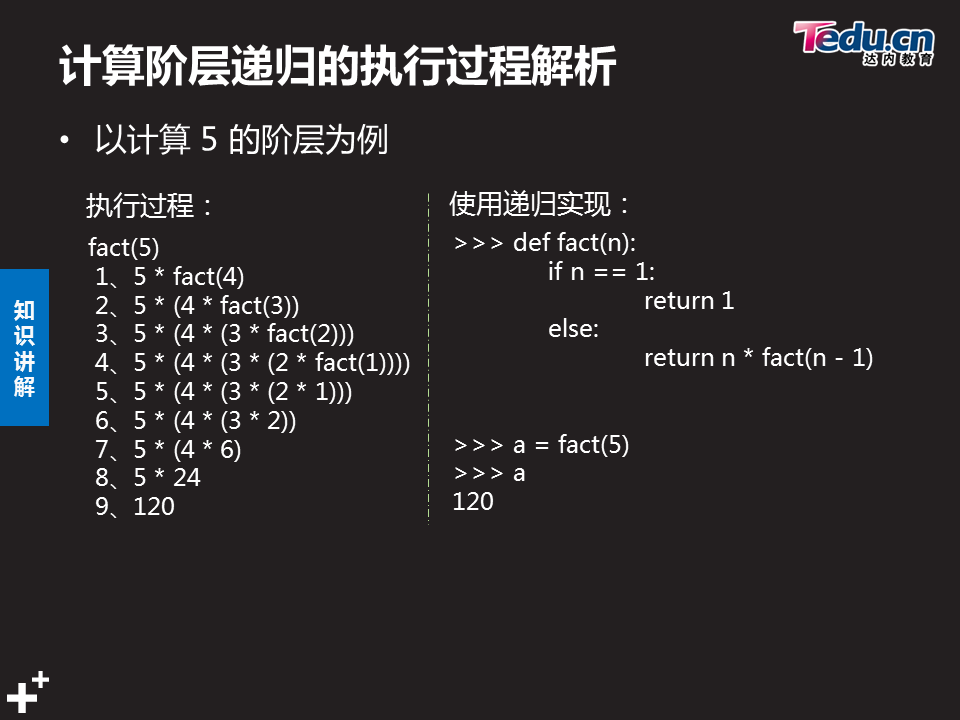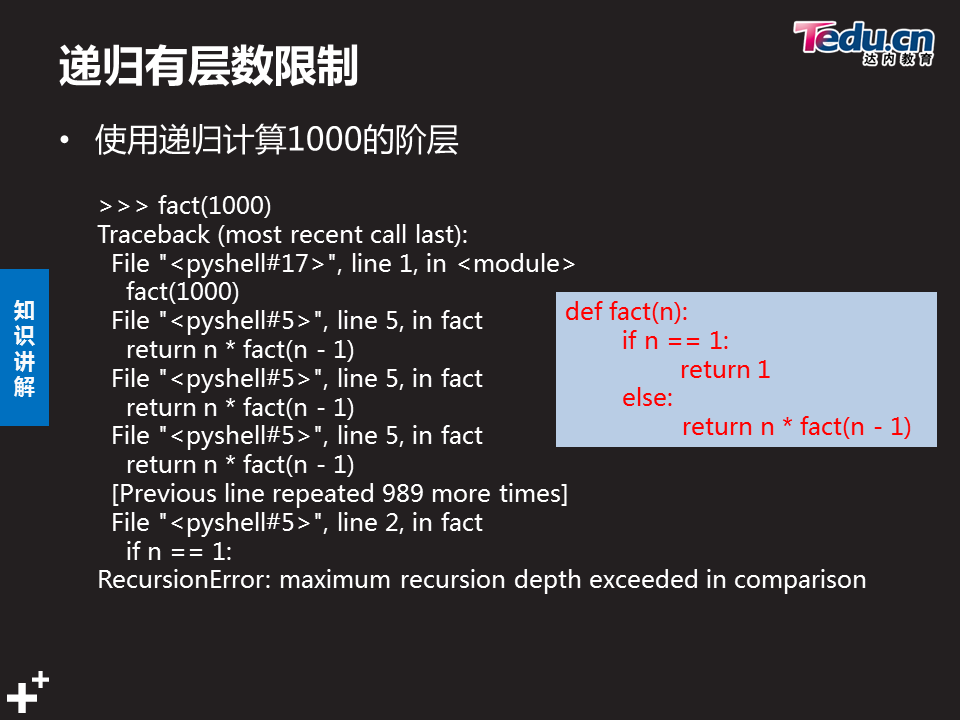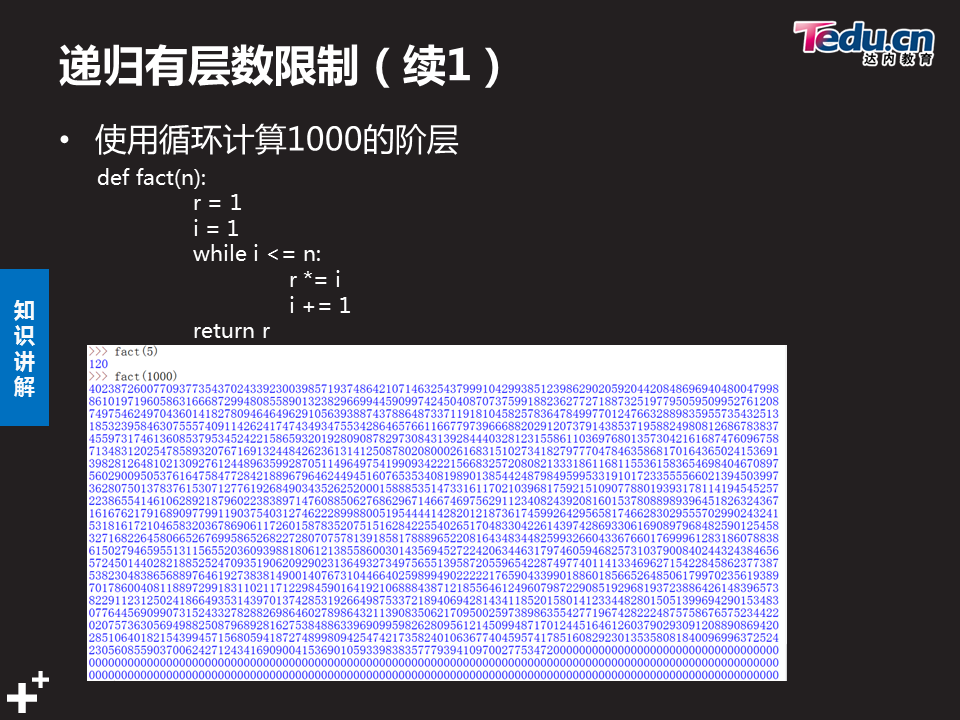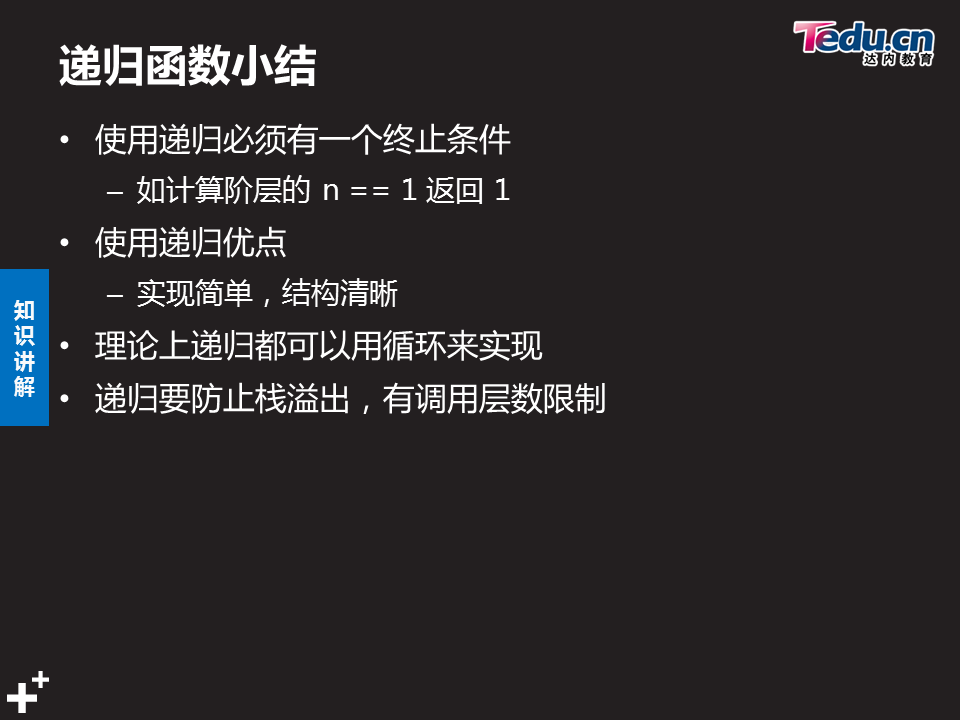递归一定要控制递归的层数，当符合条件时要终止递归
几乎所有的递归都能用while循环来代替

优点：
递归吧问题简单化，让思路更清晰，代码更简洁
缺点：
递归因系统环境影响大，当递归深时，可能会得到不可预知的结果

从原问题出发，按递归公式递推从未知到已知，最终打到递归的终止条件
回归阶段
按递归终止条件求出结果，逆向逐步代入递归公式
回归到原问题求解

闭包是引用了此函数外部的变量函数
说明：
本质上，闭包是将内部嵌套和函数外部的执行环境绑定在一起的对象
条件：
1.必须有一个内嵌函数
2.内嵌函数必须引用外部函数中的变量
3.处部函数返回值必须是内嵌函数

1的阶乘也是知道的等于1 2

5的阶乘就等于5 * 5 - 1 * 5-2 * 5-3 * 5 - 4

def fact（n）：              第一次执行函数  n = 5                     |       第二次执行这里n就变成了4                | 第三次执行这里n就变成了3

if n == 1：                 n != 1    不执行

return 1                                                          |       n != 1    不执行                        | n != 1    不执行

return n * fact（n - 1）   执行这一步 其实这里有个else

省略了 可以省略  这里n = 5                |       条件不成立 继续执行fact函数 让函数的形参| 条件不成立 继续执行fact函数 让函数的形参

5 * 函数fact（n - 1） 这里代表上面if

条件不成立 继续执行fact函数 让函数的形参  |       继续减1后继续执行                       | 继续减1后继续执行

减1后继续执行

print（fact（5））

return就是让函数结束执行并且返回一个值  说白了就是让函数变成值 变成数据

return n * fact（n - 1） 这里的n 是相等的 那么 2 * 1 然后利用这里的return继续返回 就等于 fact（n）这个函数 这时n = 2 （但是这个函数的返回值是2 * 1）

fact（n）这个函数释放 返回到调用他的函数 return n * fact（n - 1）这里  2怎么来的  n - 1 = 2  同样的道理 这里的两个n都等于3

3 * fact（3 - 1）但是呢这里的 fact（3 - 1）函数已经被释放了并return返回值  那么fact（3 - 1）等同于 2 * 1 这里就是 3 * 2 *1 然后利用这里的return返回

names = ['Tom', 'Jerry', 'Spike', 'Tyke']

排序的依据为字符串的反序:

'moT'   yrreJ    ekipS    ekyT

结果:

['Spike', 'Tyke', 'Tom', 'Jerry']

names = ['Tom', 'Jerry', 'Spike', 'Tyke']
def name(s):
return s[::-1]
print(sorted(names, key=name))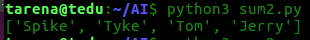# 练习：

#   1.　已知五位朋友在一起

#     第五位朋友比第四位朋友大2岁

#     第四位朋友比第三位朋友大2岁

#     第三位朋友比第二位朋友大2岁

#     第二位朋友比第一位朋友大2岁

#     第一位朋友说它10岁

#     写程序打印出第五位朋友　和第三位朋友　的年龄

def myage(n):
if n == 1:
return 10
return 2 + myage(n - 1)
print(myage(5))
print(myage(3))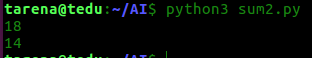4 实现带有界面的学生信息管理系统

+-------------------------+
| 1) 添加学生信息 |
| 2) 显示学生信息 |
| 3) 删除学生信息 |
| 4) 修改学生成绩 |
| q) 退出 |
+-------------------------+

# 创建输出函数：student完成    临时菜单封装
def student():
for a in range(1, 8, 2):
x = "*" * a
print(x.center(9), x.center(10), x.center(10), x.center(9))
else:
for y in range(1, 4 + 1):
c = "*"
if 4 >= 10:
c = "***"
print(c.center(9), c.center(10), c.center(10), c.center(9))
print("---------------------------------------")
print("    1):    Add Student information     ")
print("---------------------------------------")
print("    2):    print Student information   ")
print("---------------------------------------")
print("    3):    delete Student information  ")
print("---------------------------------------")
print("    4):    revamp Student information  ")
print("---------------------------------------")
print("     please input number 1~4 select    ")
print("---------------------------------------")
print("        input :(Q)Exit procedure       ")
print("---------------------------------------")
# 创建输出函数：perform完成

# 创建输入函数：input_student开始　　　　　封装信息输入

def input_student():
L = []
print("--------Student information enty--------")
while True:
if name == "":
q = input("operation succeed! return y　/　n　:")
if q == "y":
break
else:
input_student()
d = {}
d = {"name": name, "age": age, "score": score}
print("+---------------+----------+----------+")
name = d["name"].center(15)
age = d["age"].center(10)
score = d["score"].center(10)
print('''|%s|%s|%s|''' % (name, age, score))
print("+---------------+----------+----------+")
print("-----Not input Enter be no revamp!-----")
L.append(d)
return L
# 创建输入函数：input_student完成

# 创建输出函数：output_student开始　　　封装列表打印

def output_student(lst):
print("-------Student information sheet-------")
print("+---------------+----------+----------+")
print("|      Name     |    Age   |   Score  |")
print("+---------------+----------+----------+")
for d in lst:
name = d["name"].center(15)
age = d["age"].center(10)
score = d["score"].center(10)
print('''|%s|%s|%s|''' % (name, age, score))
print("+---------------+----------+----------+")
q = input("operation succeed! return y　/　n　:")
if q == "y":
pass
else:
output_student()
# 创建输出函数：output_student完成

# 创建输出函数：delete_student开始　　封装删除信息

def delete_student(lst):
name = input("please input name delete:")
for x in lst:
if x["name"] == name:
print("+---------------+----------+----------+")
name = x["name"].center(15)
age = x["age"].center(10)
score = x["score"].center(10)
print('''|%s|%s|%s|''' % (name, age, score))
print("+---------------+----------+----------+")
delet = input("whether delete (y:yes/y:no):")
if delet == "y":
lst.remove(x)
q = input("operation succeed! return y　/　n　:")
if q == "y":
pass
else:
delete_student()
# 创建输出函数：delete_student完成

# 创建输出函数：revamp_student()开始　　　封装更改信息

def revamp_student(lst):
name = input("please input name revamp:")
for x in lst:
if x["name"] == name:
print("+---------------+----------+----------+")
name = x["name"].center(15)
age = x["age"].center(10)
score = x["score"].center(10)
print('''|%s|%s|%s|''' % (name, age, score))
print("+---------------+----------+----------+")
print("-----not input Enter be no revamp!-----")
rage = input("please input age revamp:")
rscore = input("please input score revamp:")
if rage != "":
x["age"] = rage
if rscore != "":
x["score"] = rscore
print("+---------------+----------+----------+")
name = x["name"].center(15)
age = x["age"].center(10)
score = x["score"].center(10)
print('''|%s|%s|%s|''' % (name, age, score))
print("+---------------+----------+----------+")
print("-----------revamp perform!------------")
else:
print("None Error")
q = input("operation succeed! return y　/　n　:")
if q == "y":
pass
else:
revamp_student()
# 创建输出函数：revamp_student()完成

L = []
while True:
student()
if select == '1':
L += input_student()
elif select == '2':
output_student(L)
elif select == '3':
delete_student(L)
elif select == '4':
revamp_student(L)
elif select == 'q':
break

# 运行
System_menu()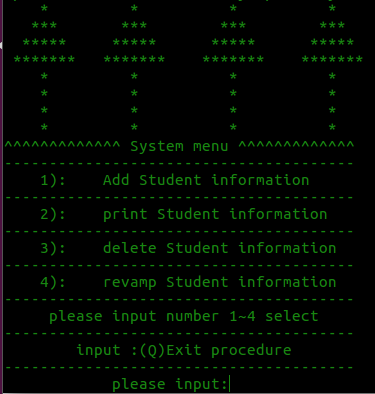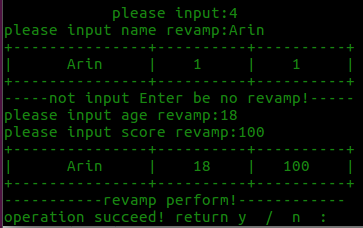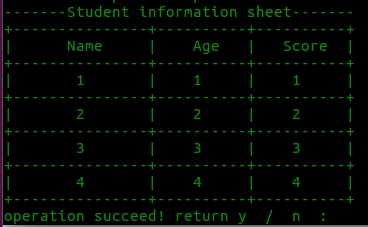0
0 收藏

### 作者的其它热门文章0 评论
0 收藏
0